# Find the 2

Find the term independent of x in the expansion of (4x3+1/2x)8

Correct result:

x =  7

#### Solution: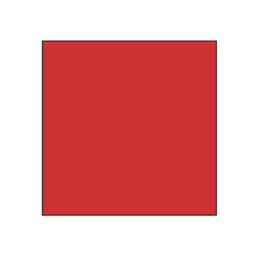We would be pleased if you find an error in the word problem, spelling mistakes, or inaccuracies and send it to us. Thank you!Tips to related online calculators
Would you like to compute count of combinations?

## Next similar math problems:

• Substitution method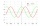Solve goniometric equation: sin4 θ - 1/cos2 θ=cos2 θ - 2
• Simplest form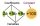Find the simplest form of the following expression: 3 to the 2nd power - 1/4 to the 2nd power
• If the 3If the 6th term of a GP is 4 and the 10th is 4/81, find common ratio r.
• Trapezoid 15Area of trapezoid is 266. What value is x if bases b1 is 2x-3, b2 is 2x+1 and height h is x+4
• What is 10What is the 5th term, if the 8th term is 80 and common ratio r =1/2?
• Non linear eqsSolve the system of non-linear equations: 3x2-3x-y=-2 -6x2-x-y=-7
• Expression with powersIf x-1/x=5, find the value of x4+1/x4
• VCP equationSolve the following equation with variations, combinations and permutations: 4 V(2,x)-3 C(2,x+ 1) - x P(2) = 0
• HP - harmonic progressionDetermine the 8th term of the harmonic progression 2, 4/3, 1,…
• What is 10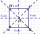What is the measure of the side of the square whose area is (4x2 + 28x+49) square units?
• Completing squareSolve the quadratic equation: m2=4m+20 using completing the square method
• Equation 23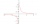Find value of unknown x in equation: x+3/x+1=5 (problem finding x)
• EllipseEllipse is expressed by equation 9x2 + 25y2 - 54x - 100y - 44 = 0. Find the length of primary and secondary axes, eccentricity, and coordinates of the center of the ellipse.
• Eq2 2Solve following equation with quadratic members and rational function: (x2+1)/(x-4) + (x2-1)/(x+3) = 23
• Common difference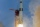The 4th term of an arithmetic progression is 6. If the sum of the 8th and 9th term is -72, find the common difference.
• Expressions 3If k(x+6)= 4x2 + 20, what is k(10)=?
• Variability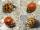In the table bellow the number of wrong produced goods in two shifts: morning shift: 2; 0; 6; 10; 2; 2; 4; 2; 5; 2; afternoon shift: 4; 4; 0; 2; 10; 2; 6; 2; 3; 10; Compare the variability in both shifts, compare the average number of wrong goods on both